Lucky Pairs (LP) is one of the names given to the non-proprietary baccarat side bet that simply pays 11-to-1 for a dealt pair. The player can wager on either or both of the Player or Banker side to be dealt a pair. This wager is also available for blackjack (player only), with varying payouts depending on the number of decks used. Shuffle tracking may provide a viable way for the AP to attack this wager in a hand-shuffled blackjack shoe game. But, as a practical matter, the blackjack version can’t be exploited using a counting system. On the other hand, baccarat provides ample opportunities for the AP. Because of the very deep placement of the cut-card, the slow pace of the game and the custom of keeping a score card, it is straight-forward for the AP to get an advantage over LP in baccarat.

To begin with, the following table gives the combinatorial analysis for LP:In particular:

• The house edge is 10.36%
• The hit frequency is 7.47%
• The standard deviation is 3.16

The mathematical analysis of this wager is quite trivial. There is only one winning situation and it’s frequency of occurrence can be directly quantified.

Let:

Nk = the number of cards of rank k left in the deck (k = 1, 2, 3, … , 13).

N = the number of cards left in the deck.

S = sum(combin(Nk,2)), k = 1, 2, 3, … , 13.

T = combin(N,2).

Then, the house edge for the Lucky Pairs bet is:

H/A = ((11S + (-1)(T – S))/T.

The player has the edge whenever the numerator is positive:

11S + (-1)(T – S) > 0

Which simplifies to:

S > (T/12)

By expanding S and T, this is equivalent to:

N1(N1 – 1) + N2(N2 – 1) + … + N13(N13 – 1) > N(N – 1)/12        (Eq. 1)

This formula is very easy to implement in computer code.

In order to estimate the edge that Eq. 1 generates, it’s important to fix the placement of the cut-card. The following is a standard method for setting the cut-card in baccarat and is the method that this analysis uses:

• The game is dealt from a shoe with 8 decks.
• At the start of each shoe, a card is exposed and burned. Based on the value of the burn card, an additional number of cards are burned but not exposed, equal to the value of the card.
• The cut-card is placed 14 cards from the end of the shoe.
• After the cut-card is dealt, one more round is dealt before shuffling.

The following table gives the results of a simulation of one hundred million (100,000,000) baccarat shoes using Eq. 1 to determine when to make the LP wager.  In this simulation, I assumed that the player made a 1-unit wager on each of the Player pair bet and Banker pair bet whenever Eq. 1 was satisfied (2 units total); otherwise no LP wager was made.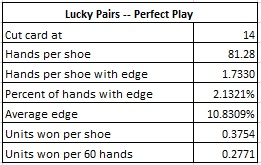We see from these results that with perfect play, the AP will make the LP wager on about 2.13% of the hands he plays, and when he makes the wager, his average edge will be about 10.83% over the house. Assume that the AP makes a LP wager of \$100 on both the Player pair and Banker pair (\$200 total) whenever he has the edge, and at no other time. Then this AP can expect to earn about \$37.54 per shoe in the long run. At a rate of 60 hands per hour, the AP will earn about \$27.71 per hour. This is a considerably higher rate of return than the Dragon Bonus bet (see this post) which returns about 0.1352 units per shoe using the optimal card counting system.

Of course, Eq. 1 is too much to handle at the tables in its full form. With a few restrictions and a bit of prior computation, however, over 87% of the potential of Eq. 1 can be realized. I call this method the “Target and Weights” technique.

To begin with, it is almost always the case that favorable opportunities against LP will occur late in the shoe. We don’t lose too much value if we restrict making the bet only when there are 60 or fewer cards remaining (N ≤ 60). For the dedicated AP, the method presented here can certainly be extended to higher N.

The next step is to compute the values of “N(N – 1)/12” for N ≤ 60. These values may be integers or fractions, but the left hand side of Eq. 1 is always an integer. We therefore define the “target” value for N to be the smallest integer greater than N(N-1)/12.

For the left hand side of Eq. 1, I also made a simplifying assumption. If there is an abundance of one rank left, then it’s almost certainly profitable to make the LP wager.  I assumed that the AP will always make the LP wager when some rank has 13 or more left (inside of 60 cards). Then the AP will only have to memorize the possible values for the terms on the left-hand-side of Eq. 1 for values from 0 to 12. The possible values that occur on the left hand side of Eq. 1 are called the “weights.”

By memorizing about 70 numbers (Targets and Weights), the LP wager can be played with great precision. The fact that baccarat allows the player to keep score means that the number of cards remaining of each rank can easily be kept. Remembering 70 values should not be tough for the dedicated AP. For example blackjack basic strategy involves remembering 550 starting configurations of two player-cards and dealer up-card.

The method for making the LP wager is easy to describe. Make the LP wager whenever:

(Sum of the Weights) ≥ Target

The following table gives the integer values corresponding to the right hand side of Eq. 1 that must be committed to memory. These are the “target” values: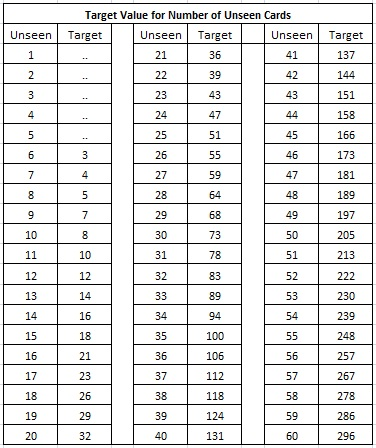The following table gives the values for the left hand side of Eq. 1 that must be committed to memory. These are the “weights” associated with the number of cards left of each rank.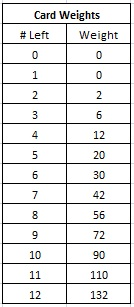A couple of examples will make this method clear. In these two examples, assume that the AP has been keeping track of the number of cards left of the various ranks. Assume there are 38 unseen cards left, so that the target is 118.

For the first example, assume the AP knows that the number of cards of each rank left in the shoe is (4,1,2,1,1,1,4,5,3,4,1,9,2). The AP recalls the weight for each of these ranks from the table above. This data is summarized in the following table: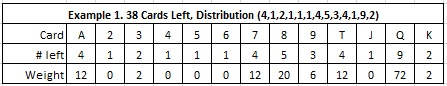The AP then sums these values. For convenience, he can start with the highest weight, and continue summing until he reaches the target of 118 (or it becomes clear that the sum will never get to 118). In this case:

72 + 20 + 12 + 12 + 12 = 128 ≥ 118

So the AP makes the LP bet in this case. In fact, the AP has an edge of 17.78% over the house with this distribution, making the LP bet a very strong play.

For the second example, assume the AP knows that the number of cards of each rank left in the shoe is (4, 2, 3, 1, 2, 2, 4, 4, 3, 4, 2, 4, 3). The AP recalls the weight for each of these ranks from the table above. This data is summarized in the following table: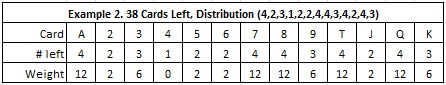The AP then sums these values. It soon becomes clear that there is no way this sum will get to the target value of 118. The sum of all the weights is 86 < 118.

So the AP does not make the LP bet in this case. In fact, the House has an edge of 26.60% over the player with this distribution, making the LP bet a very bad play in this situation.

After doing a few examples, it is easy to get a good intuition for advantageous situations. An edge requires a strong imbalance in the rank distribution with at least one large weight.

The following table gives the results of a simulation of one hundred million (100,000,000)  baccarat shoes using the target and weights method. In this simulation, I assumed that the player made a one unit wager on each of the Player pair bet and Banker pair bet whenever Eq. 1 was satisfied (2 units total); otherwise no LP wager was made.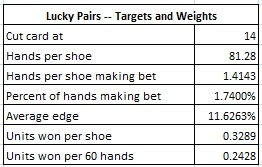We see from these results that using Targets and Weights, the AP will make the LP wager on about 1.74% of the hands he plays, and when he makes the LP wager, his average edge will be about 11.63% over the house. Assume that the AP makes a LP wager of \$100 on both the Player pair and Banker pair (\$200 total) whenever he has the edge, and at no other time. Then this AP can expect to earn about \$32.89 per shoe in the long run (\$24.28 per hour). This is compared to the optimal value of \$37.54 per shoe (\$27.71 per hour). It follows that the Target and Weights method yields over 87% of the return from perfect play.

The main defense against exploiting the LP wager is cut-card placement. This turns out to be a powerful remedy for the casino. The following table gives statistical results for cut-card placements from 12 to 26 cards using the Target and Weights method. Each line of data was obtained by a simulation of twenty million (20,000,000) shoes.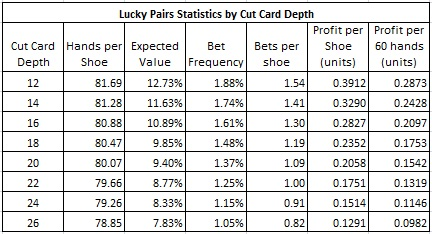The LP side bet has been around for a long time.  Clearly APs have done this work before. From what I can tell, no serious effort has previously been put into quantifying its vulnerability from the casino side. Fortunately for the casino side, game protection is easy.

The following are my suggestions for safeguarding LP from advantage play:

• Increase the cut-card depth to at least 26 cards.
• Do not expose the initial burn cards.
• Watch for players who make the LP wager late in the shoe, who bet on both sides, and who make table maximum wagers.
• Watch for players who keep score in a manner that indicates rank tracking.
• Watch for players who scratch mathematical sums on their score cards.
• Watch out for exploiting weak hand-shuffles through shuffle tracking.

Received his Ph.D. in Mathematics from the University of Arizona in 1983. Eliot has been a Professor of both Mathematics and Computer Science. Eliot retired from academia in 2009. Eliot Jacobson